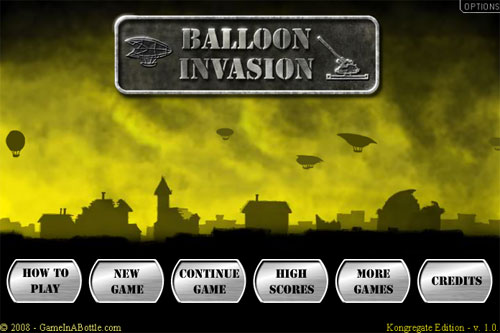# Flash prototype of a game like Balloon Invasion

One of the latest successful games released last week was Balloon Invasion.It’s a mix between an artillery and a defense game, with a World War II theme.

I made a little prototype of the game, that will be explained another day. It’s nothing so interesting, just a mix between Create a flash artillery game and Managing multiple collision detection with Flash tutorials.

That’s the good point when you write a lot of code: the more you write, the more you can recycle.

The main difference however is the firing system: enemies don’t get hurt by bullets but mby explosions bullets make when they come to the spot you fired on… just like “real” artillery

```Mouse.hide();
attachMovie("crosshair","crosshair",1);
attachMovie("tank","tank",2,{_x:450, _y:350});
fire_array = new Array();
fire_delay = 30;
just_fired = 0;
crosshair.onEnterFrame = function() {
this._x = _xmouse;
this._y = _ymouse;
};
tank.onEnterFrame = function() {
mousex = _xmouse-this._x;
mousey = (_ymouse-this._y)*-1;
angle = Math.atan(mousey/mousex)/(Math.PI/180);
if (mousex<0) {
angle += 180;
}
if (mousex>=0 && mousey<0) {
angle += 360;
}
if (angle>160) {
angle = 160;
}
if (angle<20) {
angle = 20;
}
firepower = Math.sqrt(mousex*mousex+mousey*mousey);
if (firepower>200) {
firepower = 200;
}
this.cannon._rotation = angle*-1;
};
function onMouseDown() {
if (just_fired>fire_delay) {
just_fired = 0;
angle = tank.cannon._rotation;
start_ball_x = tank._x+48*Math.cos(angle*Math.PI/180);
start_ball_y = tank._y+48*Math.sin(angle*Math.PI/180);
cannonball_fired = attachMovie("cannonball", "cannonball_"+_root.getNextHighestDepth(), _root.getNextHighestDepth(), {_x:start_ball_x, _y:start_ball_y});
cannonball_fired.dirx = Math.cos(angle*Math.PI/180)*firepower;
cannonball_fired.diry = Math.sin(angle*Math.PI/180)*firepower;
cannonball_fired.fire_coord_x = _root._xmouse;
cannonball_fired.fire_coord_y = _root._ymouse;
cannonball_fired.onEnterFrame = function() {
this._x += this.dirx/50;
this._y += this.diry/50;
dist_x = this._x-this.fire_coord_x;
dist_y = this._y-this.fire_coord_y;
dist = Math.sqrt(dist_x*dist_x+dist_y*dist_y);
if (dist<20) {
fire_array.push("explosion"+_root.getNextHighestDepth());
exp = attachMovie("explosion", "explosion"+_root.getNextHighestDepth(), _root.getNextHighestDepth(), {_x:this._x, _y:this._y});
exp.onEnterFrame = function() {
this._width += 2;
this._height += 2;
this._alpha -= 2;
if (this._alpha<0) {
for (x in fire_array) {
if (this.name == _root[fire_array[x]].name) {
fire_array.splice(x,1);
}
}
this.removeMovieClip();
}
};
this.removeMovieClip();
}
};
}
}
_root.onEnterFrame = function() {
just_fired++;
new_enemy = Math.random()*100;
if (new_enemy<1) {
enemy = attachMovie("enemy", "enemy"+_root.getNextHighestDepth(), _root.getNextHighestDepth(), {_x:-40, _y:Math.random()*200+50});
enemy.speed = Math.random()*2+1;
enemy.onEnterFrame = function() {
this._x += this.speed;
if (this._x>550) {
this.removeMovieClip();
}
for (x in fire_array) {
dist_x = this._x-_root[fire_array[x]]._x;
dist_y = this._y-_root[fire_array[x]]._y;
dist = Math.sqrt(dist_x*dist_x+dist_y*dist_y);
if (dist<(this._width+_root[fire_array[x]]._width)/2) {
this._alpha -= 5;
if (this._alpha<0) {
this.removeMovieClip();
}
}
}

};
}
};```

And here's the result:

214 GAME PROTOTYPES EXPLAINED WITH SOURCE CODE
// 1+2=3
// 10000000
// 2 Cars
// 2048
// Avoider
// Ballz
// Block it
// Blockage
// Bloons
// Boids
// Bombuzal
// Breakout
// Bricks
// Columns
// CubesOut
// Dots
// DROP'd
// Dudeski
// Eskiv
// Filler
// Fling
// Globe
// HookPod
// Hundreds
// InkTd
// Iromeku
// Lumines
// Magick
// MagOrMin
// Maze
// Memdot
// Nano War
// Nodes
// o:anquan
// Ononmin
// Pacco
// Phyballs
// Platform
// Poker
// Pool
// Poux
// Pudi
// qomp
// Racing
// Renju
// SameGame
// Security
// Sling
// Slingy
// Sokoban
// Splitter
// Sproing
// Stack
// Stringy
// Sudoku
// Tetris
// Threes
// Toony
// Turn
// TwinSpin
// vvvvvv
// Wordle
// Worms
// Yanga
// Zhed
// zNumbers